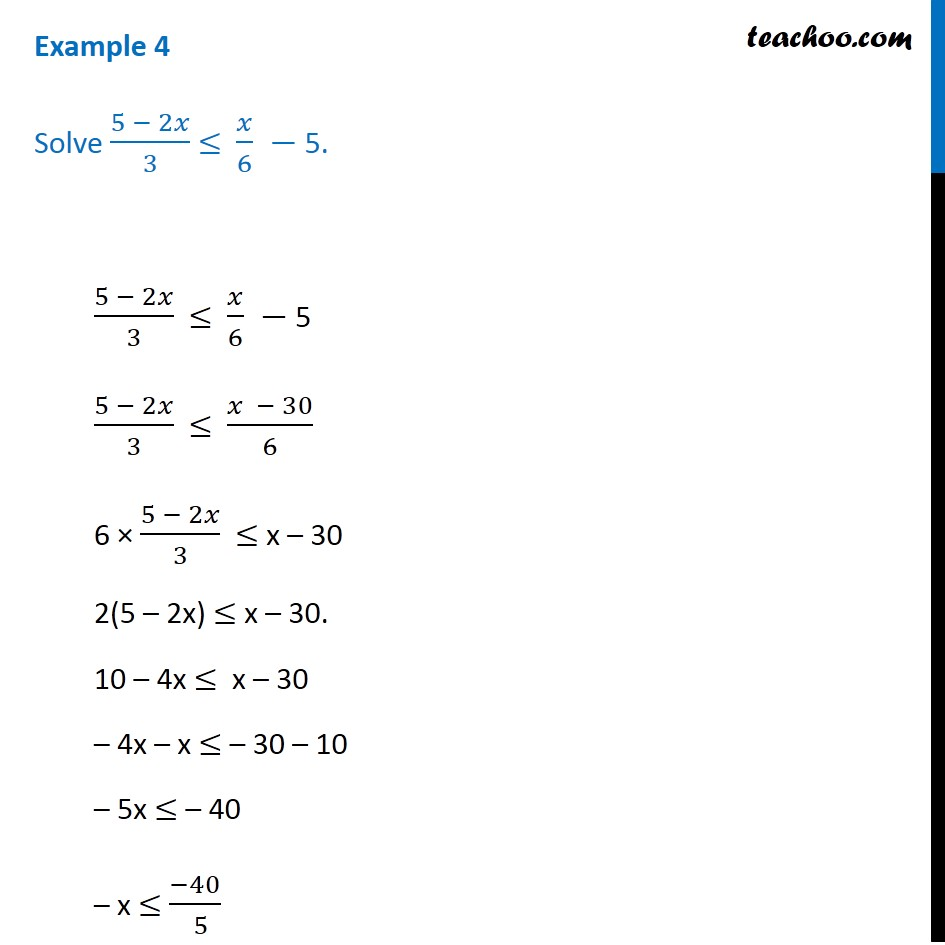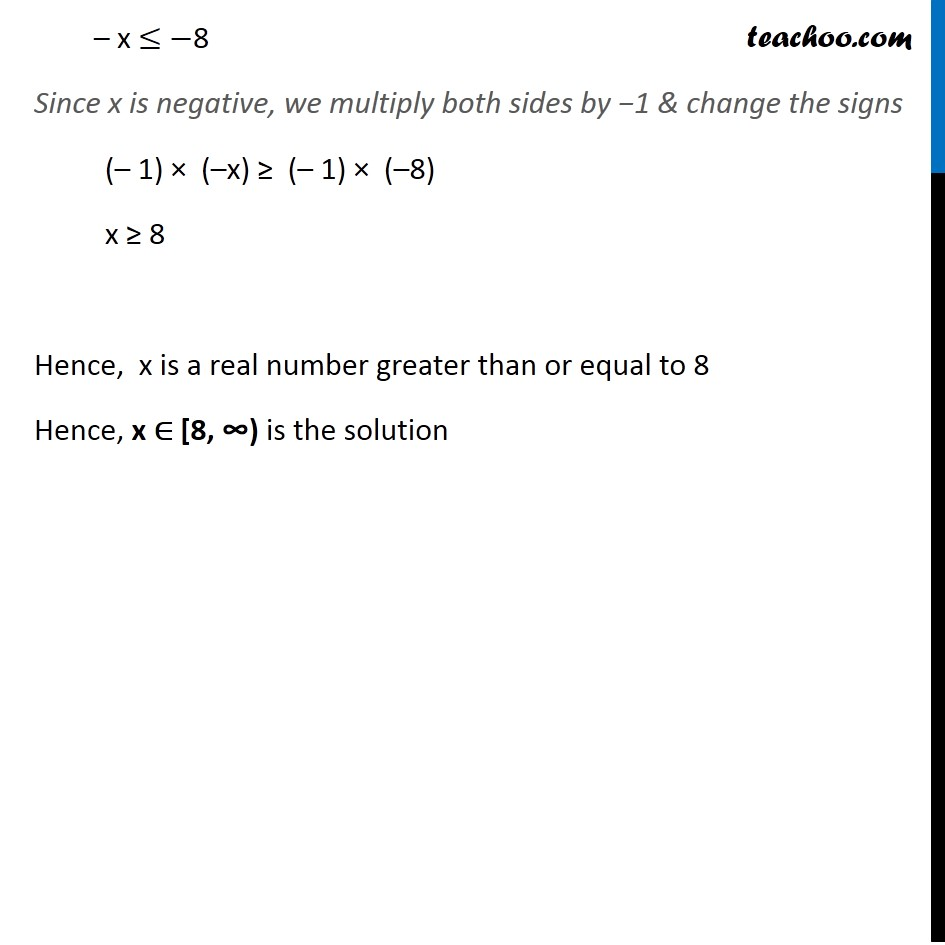Examples

Chapter 5 Class 11 Linear Inequalities
Serial order wiseLearn in your speed, with individual attention - Teachoo Maths 1-on-1 Class

### Transcript

Example 4 Solve (5 − 2𝑥)/3 ≤ 𝑥/6 − 5. (5 − 2𝑥)/3 ≤ 𝑥/6 − 5 (5 − 2𝑥)/3 ≤ (𝑥 − 30)/6 6 × (5 − 2𝑥)/3 ≤ x – 30 2(5 – 2x) ≤ x – 30. 10 – 4x ≤ x – 30 – 4x – x ≤ – 30 – 10 – 5x ≤ – 40 – x ≤ (−40)/( 5) – x ≤ −8 Since x is negative, we multiply both sides by −1 & change the signs (– 1) × (–x) ≥ (– 1) × (–8) x ≥ 8 Hence, x is a real number greater than or equal to 8 Hence, x ∈ [8, ∞) is the solution